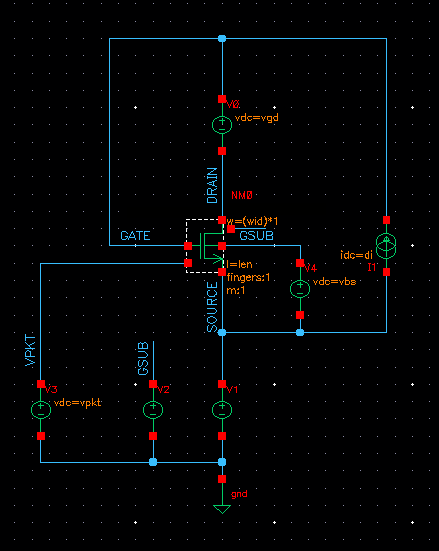# Getting gm by Id vs Id by W by L graphs in CadenceThe following steps need to be done for getting the gm/Id vs Id/(W/L) graphs in Cadence for a MOS transistor.

# Create the schematic [top]

The schematic is a simple transistor schematic. The schematic below shows an example:The above schematic shows that the drain current(di), vgd, vbs, W and L are parameterized and they are sweeped in the simulation script (except vbs) to get the graphs for a wide range of conditions and check the variation of the graph for them.

# Save Operating Point Info [top]

Create a text file called mysaves_t18.scs and add the following line in it:

save MN0_PSP.m0:oppoint


Note that the .m0 is only needed because this model is implemented as s Sub Circuit in the models file. If the model is not a subcircuit and something direct like a BSIM model then .m0 would not be needed.

# Setup simulation in ADE [top]

Setup the DC sweep simulation in ADE by DC sweeping di from a low value to a high value for a typical vgd, W and L. Set the sweep to be logarithmic with 50 points per decade. Also setup expressions to calculate
1. gm/Id = gm/di expression: (getData("MNO_PSP.m0:gm" ?result "dc-dc")/IS("/V1/PLUS"))
2. Id/(W/L) expression: (IS("/V1/PLUS")/(5e-6/180e-9))

Run the Simulation and see the results.

# Create the Ocean Script [top]

Save the Ocean Script from the ADE environment and modify it to look like the following:

; Select the waveform tool
ocnWaveformTool( 'wavescan )

; Select the simulator
simulator( 'spectre )

; Specify the Design Netlist
design(	 "/sim/mgupta/nch_char_1/spectre/schematic/netlist/netlist")

; Specify the Results Directory
resultsDir( "/sim/mgupta/nch_char_1/spectre/schematic" )

; Specify the model files set
modelFile(
)

; Specify the Definition file created to save the operating point
definitionFile(
)

; Convert the main run steps to a procedure 'mysim' and convert width, length, vgd, istart, istop
; etc as variables to be passed along with the filehandle where to write the results
procedure(mysim(istart istop length width vgd fileHandle)
analysis('dc ?param "di"  ?start istart  ?stop istop  ?dec "50"  )
desVar(	  "l" length	)
desVar(	  "vgd" vgd	)
desVar(	  "di" 5u	)
desVar(	  "w" width	)
save( 'i "/V1/PLUS" )
temp( 27 )
run()
gm_by_Ids = (getData("MN0_PSP.m0:gm" ?result "dc-dc") / IS("/V1/PLUS"))
id_by_w_l = IS("/V1/PLUS")*length/width

;Comment out the plot statement
;plot( gm_by_Ids ?expr '( "gm_by_Ids" ) )

; Write the results in the filehandle
ocnPrint( ?output fileHandle ?numberNotation 'engineering ?numSpaces 10 id_by_w_l gm_by_Ids)
)

; Create file for writing the results
resultFile = outfile( "./Curves/gmByIdCurves.csv" "w")
; Close the file
close(resultFile)

; Lists for iterating the simulation

length_list = list(180e-9 500e-9 1e-6 1.5e-6 2e-6 2.5e-6 3e-6 3.5e-6 4e-6 4.5e-6 5e-6 5.5e-6 6e-6 6.5e-6 7e-6 7.5e-6 8e-6 8.5e-6 9e-6 9.5e-6 10e-6)
;length_list = list(180e-9 500e-9)
width_list = list(2e-6 4e-6 6e-6 8e-6 10e-6 15e-6 20e-6)
;width_list = list(2e-6 4e-6)
vgd_list = list(0.3 0 -0.3 -0.6 -0.9)
;vgd_list = list(0.3 0)

min_IdbyWL = 1e-9
max_IdbyWL = 200e-6

; Loop through all the lists
foreach( vgd vgd_list
foreach( width width_list
foreach(length length_list
; Calculate istart, istop so that Id/(W/L) starts and stops the same for every W/L combination
istart = min_IdbyWL*width/length
istop = max_IdbyWL*width/length
; Write the data in a temp File
tempFile = outfile("./Curves/gmByIdtmp.out" "w")
mysim(istart istop length width vgd tempFile)
; Close the file
close(tempFile)
; Combine the data file and temp file to an intermediate file
; Create the intermediate file
system(sprintf(nil "pr -mr -t -s\\  %s %s > %s" "./Curves/gmByIdCurves.csv" "./Curves/gmByIdtmp.out" "./Curves/gmByIdinter.out"))
; remove the Curves data file
system(sprintf(nil "rm -rf ./Curves/gmByIdCurves.csv"))
; rename intermediate file to Curves file
system(sprintf(nil "mv ./Curves/gmByIdinter.out ./Curves/gmByIdCurves.csv"))
)
)
)

; Remove the temporary File
system(sprintf(nil "rm -rf ./Curves/gmByIdtmp.out"))


Save this ocean script (say 'gmByIdScript.ocn') and load it from the CIW window by typing:

load("gmByIdScript.ocn")


This would start the simulations and at the end we would have the file gmByIdCurves.csv with all the simulation results.

# Draw the Graph using MATLAB [top]

Finally create the following script in MATLAB and name it mergeGraphs.m and run it to create the graphs in a figure:
% Script to read gmbyId.txt and merge the readings to just 1 graph
% 1st column is taken as Current, 2nd column as Id/(W/L), 3rd column as
% gm/Id
% Remove the 1st 2 lines as the header lines

figure(1);
for count = 2:3:size(arr,2)
semilogx(arr([1:size(arr,1)],count),arr([1:size(arr,1)],count+1),'r');
hold on;
end
grid on;

xlabel('Id/(W/L)')
ylabel('gm/Id')
title('gm/Id vs Id/(W/L) for 2V NMOS TSMC 0.18\mum RF; 180nm \leq L \leq 10\mum, 2\mum \leq W \leq 20\mum, 0.3V \leq Vgd \leq -0.9V')


# Drawing the Graph using gsl-shell [top]

Use the following script. The script can be loaded in gsl-shell using the command dofile('script_path_and_name').
csv = require 'csv'
-- Convert it to a 2D numeric array since the csv is space separated and not "," separated
curves = {}
for i = 4,#t do
curves[#curves+1] = {}
for n in string.gmatch(t[i],"%S+") do
curves[#curves][#curves[#curves]+1] = tonumber(n)
end
end

-- Now all the data table is in Curves:
-- 1st column is drain current
-- 2nd column is Id/(W/L)
-- 3rd column is gm/Id
-- And it repeats
p = graph.plot()

for i = 2,#curves,3 do
-- Create the line here
ln = graph.path(math.log10(curves[i]),curves[i+1])
for j = 2,#curves do
ln:line_to(math.log10(curves[j][i]),curves[j][i+1])
end
end
p:show()


## Sample Graph [top]

Shown below is a sample graph from the above steps:NOTE This graph was taken with a linear sweep a better graph will result of the sweep is set to logarithmic especially with no discontinuity in the slope as it seems in this one.